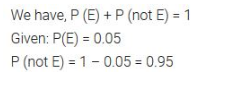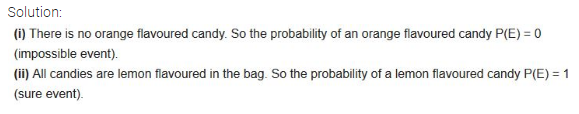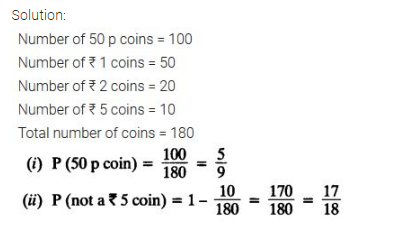• +91 9971497814
• info@interviewmaterial.com

# Probability Ex-15.1 Interview Questions Answers

### Related Subjects

Question 1 : Complete the following statements:

(i) Probability ofan event E + Probability of the event ‘not E’ = ___________.

(ii) The probabilityof an event that cannot happen is __________. Such an event is called ________.

(iii) Theprobability of an event that is certain to happen is _________. Such an eventis called _________.

(iv) The sum of theprobabilities of all the elementary events of an experiment is __________.

(v) The probabilityof an event is greater than or equal to and less than or equal to __________.

Solution:

(i) Probability of an event E + Probability ofthe event ‘not E’ = 1.

(ii) The probability of an event that cannothappen is 0. Such an event is called an impossible event.

(iii) The probability of an event that iscertain to happen is 1. Such an event is called a sure or certainevent.

(iv) The sum of the probabilities of all theelementary events of an experiment is 1.

(v) The probability of an event is greaterthan or equal to 0 and less than or equal to 1.

Question 2 : Which of the following experiments have equally likely outcomes? Explain.

(i) A driverattempts to start a car. The car starts or does not start.

(ii) A playerattempts to shoot a basketball. She/he shoots or misses the shot.

(iii) A trial ismade to Solution: a true-false question. The Solution: is right or wrong.

(iv) A baby is born.It is a boy or a girl.

Solution:

(i) This statement does not have equallylikely outcomes as the car may or may not start depending upon various factorslike fuel, etc.

(ii) Even this statement does not have equallylikely outcomes as the player may shoot or miss the shot.

(iii) This statement has equally likelyoutcomes as it is known that the solution is either right or wrong.

(iv) This statement also has equally likelyoutcomes as it is known that the newly born baby can either be a boy or a girl.

Question 3 : Why is tossing a coin considered to be a fairway of deciding which team should get the ball at the beginning of a footballgame?

Tossing of a coin is a fair way of decidingbecause the number of possible outcomes are only 2 i.e. either head or tail.Since these two outcomes are an equally likely outcome, tossing isunpredictable and is considered to be completely unbiased.

Question 4 :

Which of thefollowing cannot be the probability of an event?

(A) 2/3 (B) -1.5 (C)15% (D) 0.7

The probability of any event (E) always liesbetween 0 and 1 i.e. 0 ≤ P(E) ≤ 1. So, from the above options, option (B) -1.5cannot be the probability of an event.

Question 5 :

If P(E) = 0.05, whatis the probability of ‘not E’?

Answer 5 :Question 6 :

A bag contains lemonflavored candies only. Malini takes out one candy without looking into the bag.

What is the probability that she takes out

(i) an orange flavored candy?

(ii) a lemon flavored candy?Question 7 :

It is given that ina group of 3 students, the probability of 2 students not having the samebirthday is 0.992. What is the probability that the 2 students have the samebirthday?

Let the event wherein 2 students having thesame birthday be E

Given, P(E) = 0.992

We know,

P(E)+P(not E) = 1

Or, P(not E) = 1–0.992 = 0.008

The probability that the 2 students have the same birthday is0.008

Question 8 :

A bag contains 3 redballs and 5 black balls. A ball is drawn at random from the bag.

What is the probability that the ball drawn is

(i) red?

(ii) not red?

Solution

The total number of balls = No. of red balls +No. of black balls

So, the total no. of balls = 5+3 = 8

We know that the probability of an event isthe ratio between the no. of favourable outcomes and the total number ofoutcomes.

P(E) = (Number of favourable outcomes/ Totalnumber of outcomes)

(i) Probability of drawing red balls = P (redballs) = (no. of red balls/total no. of balls) = 3/8

(ii) Probability of drawing black balls = P(black balls) = (no. of black balls/total no. of balls) = 5/8

Question 9 :

A box contains 5 redmarbles, 8 white marbles and 4 green marbles. One marble is taken out of thebox at random.

What is the probability that the marble taken out will be

(i) red?

(ii) white?

(iii) not green?

Solution

The Total no. of balls = 5+8+4 = 17

P(E) = (Number of favourable outcomes/ Totalnumber of outcomes)

(i) Total number of red balls = 5

P (red ball) = 5/17 = 0.29

(ii) Total number of white balls = 8

P (white ball) = 8/17 = 0.47

(iii) Total number of green balls = 4

P (green ball) = 4/17 = 0.23

P (not green) = 1-P(green ball) = 1-(4/7) = 0.77

Question 10 :

A piggy bankcontains hundred 50p coins, fifty ₹1 coins, twenty ₹2 coins and ten ₹5 coins.

If it is equally likely that one of the coins will fall out when the bank is turned upside down, what is the probability that the coin

(i) will be a 50 p coin?

(ii) will not be a ₹5 coin?Todays Deals### Probability Ex-15.1 Contributorskrishan

Name:
Email:

# Latest News# 9000 interview questions in different categories